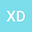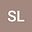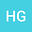loading page

LT-SCM-SNI method for solving definite solution problems of three-interval composite nonlinear partial differential equations and its application
•••• +3
• Xiaoxu Dong,
• Shun Li,
• Hong Guo,
• Wei Li,
• Wenjing Li,
• Qun Liu
Xiaoxu Dong
Xihua University
Author ProfileShun Li
Xihua University
Author ProfileHong Guo
Xihua University
Author ProfileWei Li
Xihua University
Author ProfileWenjing Li
Xihua University
Author ProfileQun Liu
Xihua University
Author Profile## Abstract

The definite solution problems of three-interval composite nonlinear partial differential equations (PDE) under different conditions is studied in the paper. Then the definite solution problems are solved by Laplace transformation - similar constructing method - Stehfest numerical inversion equation (LT-SCM-SNI method). Firstly, the definite solution problems of three-interval composite nonlinear PDE is transformed into the boundary value problems of three-interval composite linear of ordinary differential equation (ODE) by linearization and Laplace transformation (LT). Secondly, the solution in Laplace space of the boundary value problems of three-interval composite linear of ODE is obtained through using the similar constructing method (SCM). The solution in real space of the definite solution problems of three-interval composite nonlinear PDE is obtained through using the Stehfest numerical inversion equation (SNI) and linearization equation. Finally, the nonlinear spherical seepage model of three-interval composite reservoir under infinite outer boundary conditions is solved through using the LT-SCM-SNI method. The influence of various parameters on dimensionless bottom-hole transient pressure is studied. The example shows the simplicity and practicability of the method presented in the paper.